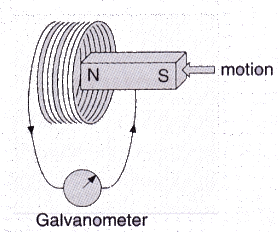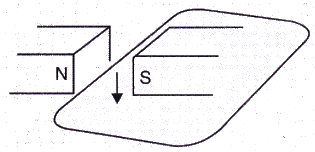Courses

# Solutions of Magnetic Effects of Electric Current (Page No-105) - Physics By Lakhmir Singh, Class 10 Class 10 Notes | EduRev

## Class 10 Physics Solutions By Lakhmir Singh & Manjit Kaur

Created by: Ajay Batra

## Class 10 : Solutions of Magnetic Effects of Electric Current (Page No-105) - Physics By Lakhmir Singh, Class 10 Class 10 Notes | EduRev

The document Solutions of Magnetic Effects of Electric Current (Page No-105) - Physics By Lakhmir Singh, Class 10 Class 10 Notes | EduRev is a part of the Class 10 Course Class 10 Physics Solutions By Lakhmir Singh & Manjit Kaur.
All you need of Class 10 at this link: Class 10

Lakhmir Singh Physics Class 10 Solutions Page No:105

Question 37: A coil is connected to a galvanometer. When the N-pole of a magnet is pushed into the coil, the galvanometer deflected to the right. What deflection, if any, is observed when :
(a) the N-pole is removed ?
(b) the S-pole is inserted ?
(c) the magnet is at rest in the coil ?
State three ways of increasing the deflection on the galvanometer.

Solution : (a) The galvanometer deflects to the left.
(b) The galvanometer deflects to the left.
(c) No deflection in galvanometer.
The deflection in the galvanometer can be increased by
i. increasing the number of turns in the coil
i. using a strong magnet
ii. increasing the speed with which magnet is moved in the coil.

Question 38: When the magnet shown in the diagram below is moving towards the coil, the galvanometer gives a reading to the right.
(i) What is the name of the effect being produced by the moving magnet ?
(ii) State what happens to the reading shown on the galvanometer when the magnet is moving away from the coil.
(iii) The original experiment is repeated. This time the magnet is moved towards the coil at a great speed. State two changes you would notice in the reading on the galvanometer.Solution : (i) Electromagnetic induction.
(ii) The galvanometer gives a reading to the left.
(iii) Large deflection to right occurs more quickly.

Question 39: If you hold a coil of wire next to a magnet, no current will flow in the coil. What else is needed to induce a current ?

Solution : Relative motion between the coil and the magnet.

Question 40: The wire in Figure below is being moved downwards through the magnetic field so as to produce induced current.
What would be the effect of :
(a) moving the wire at a higher speed ?
(b) moving the wire upwards rather than downwards ?
(c) using a stronger magnet ?
(d) holding the wire still in the magnetic field ?
(e) moving the wire parallel to the magnetic field lines ?Solution : (a) Current increased.
(b) Current reversed.
(c) Current increased.
(d) Zero current.
(e) Zero current.

Question 41: Two coils A and B of insulated wire are kept close to each other. Coil A is connected to a galvanometer while coil B is connected to a battery through a key. What would happen if :
(i) a current is passed through coil B by plugging the key ?
(ii) the current is stopped by removing the plug from the key ?

Solution : (i) Galvanometer pointer moves to one side showing that a momentary current is induced in the coil A.
(ii) Galvanometer pointer moves to the other side showing that the direction of momentarily induced current has been reversed.
The phenomenon taking place here is electromagnetic induction. When the current is passed through coil B or is stopped, the magnetic field linked with coil A changes due to which an induced current is produced in the coil.

Question 42: A portable radio has a built-in transformer so that it can. work from the mains instead of batteries. Is this a step-up or step down transformer ?

Solution : Step-down transformer (which reduces the voltage).

Offer running on EduRev: Apply code STAYHOME200 to get INR 200 off on our premium plan EduRev Infinity!

94 docs

,

,

,

,

,

,

,

,

,

,

,

,

,

,

,

,

,

,

,

,

,

,

,

,

;